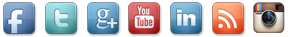### International Publication :: Physical Education

 Title: Fuzzy theta-perfect irreducible mappings and fuzzy theta-proximity spaces Authors: KANDIL, A (KANDIL, A); KERRE, EE (KERRE, EE); NOUH, AA (NOUH, AA); ELSHAFEI, ME (ELSHAFEI, ME) Full Text From Publiser In this paper we introduce and study the concepts of fuzzy perfect, fuzzy theta-continuous, fuzzy irreducible and fuzzy theta-perfect mappings. It is shown that the small image of a canonical open fuzzy set remains canonical open under fuzzy theta-closed irreducible mapping. Also, we introduce and study the concept of fuzzy theta-proximity spaces and point out their interrelation with separated fuzzy proximity spaces by means of fuzzy theta-perfect irreducible mappings. Title: Application of he's parameter-expansion method for the nonlinear differential equations Authors: Sweilam, NH (Sweilam, N. H.)[ 1 ] ; Khader, MM (Khader, M. M.)[ 2 ] Full Text From Publiser In this paper, the parameter-expansion method is implemented to a non-linear differential equation and a coupled system of non-linear partial differential equations. One iteration is sufficient to obtain a highly accurate solution, which is valid for the whole solution domain. Comparison of the obtained solution with the exact one shows that the method is very effective and convenient. The accuracy of this method is verified by the focusing Manakov systems by ensuring that the conserved quantities remain almost constant.